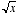# square root

Square Root

A nonnegative number that must be multiplied times itself to equal a given number. The square root of x is writtenor x½. For example,since 32 = 9.

Note:never refers to a negative number. Even though (–3)(–3) = 9, we do not say that –3 is a value of. Also, if x itself is negative thenis imaginary.